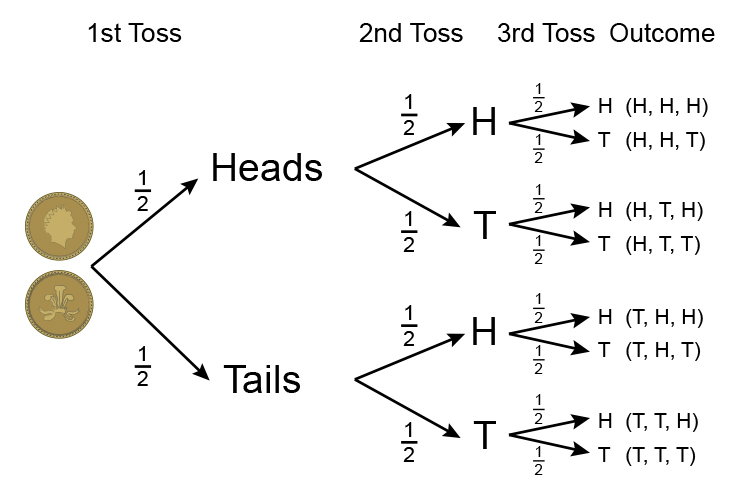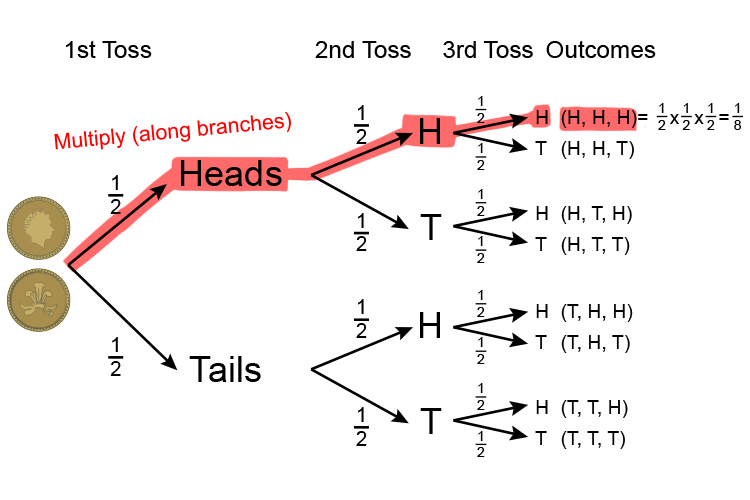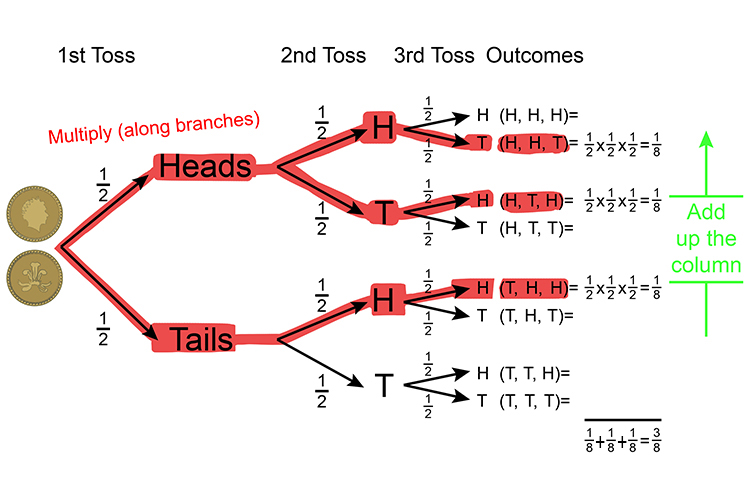# Probability tree examples

Example 1

What is the probability of a coin being tossed and getting 3 heads in a row?

A classic probability tree - Remember that tossing one coin gives:

Probability\=(Right)/(All) =(The\  \n\umber\ of\ ways\ of \ ac\hiev\i\ng\ suc\ess)/(T\he\ \t\otal\ n\umber\ of \ possibl\e\ outcccomes

Heads\ortails=1/2\ \overset{(Right)}{\underset{(All\ possibilites)}{text}} ]

A probability tree diagram which represents a coin being tossed three times is:P(H,H,H)=1/2\times\1/2\times\1/2=1/8

Probability = 1 in 8 or = 0.125

And if 1 = 100%   then 0.125=12.5% chance

The probability of a coin being tossed and getting 3 heads in a row is 0.125.

Example 2

What is the probability of a coin being tossed three times and getting 2 heads and a tail in any combination?

Remember that tossing one coin gives:

Probability\=(Right)/(All) =(The\  \n\umber\ of\ ways\ of \ ac\hiev\i\ng\ suc\ess)/(T\he\ \t\otal\ n\umber\ of \ possibl\e\ outcccomes

Heads\ or tails=1/2\ \overset{(Right)}{\underset{(All\ possibilites)}{text}} ]

A probability tree diagram which represents a coin being tossed three times is:Probability (2 Heads and a tail in any combination) = 1/8+1/8+1/8=3/8=0.375

And if 1 = 100%             then 0.375=37.5% chance